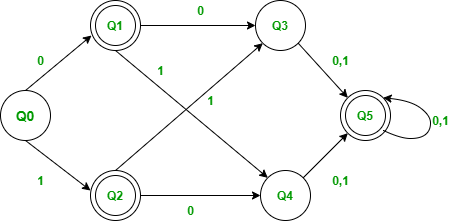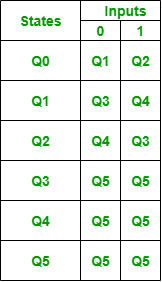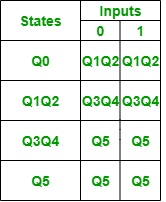# Minimization of DFA using Myhill-Nerode Theorem

• Difficulty Level : Easy
• Last Updated : 08 Oct, 2021

Minimization of DFA using Myhill-Nerode Theorem :
Minimization of DFA is Required to obtain the minimal and equivalent version of any DFA which consists of minimum number of states possible. Myhill-Nerode theorem can be used to convert a DFA to its equivalent DFA with minimum no of states. This method of minimization is also called Table filling method. There is also another method called Partitioning Method or Equivalence Method for the minimization of DFA ( please visit  https://www.geeksforgeeks.org/minimization-of-dfa/ to know about Equivalence/Partitioning Method).

#### Steps for the Minimization of DFA :

1. Create the pairs of all the states involved in the given DFA.
2. Mark all the pairs (Qa,Qb) such a that Qa  is Final state and Qb is Non-Final State.
3. If there is any unmarked pair (Qa,Qb) such a that δ(Qa,x) and δ(Qb,x) is marked, then mark (Qa,Qb). Here x is a input symbol. Repeat this step until no more marking can be made.
4. Combine all the unmarked pairs and make them a single state in the minimized DFA.

#### Example

Consider the following DFA,Following is the transition table for the above DFAMinimizing the above DFA using Myhill-Nerode Theorem :

Step-1: Create the pairs of all the states involved in DFA.Step-2: Mark all the pairs (Qa,Qb) such a that Qa  is Final state and Qb is Non-Final State.Step-3: If there is any unmarked pair (Qa,Qb) such a that δ(Qa,x) and δ(Qb,x) is marked, then mark (Qa,Qb). Here x is a input symbol. Repeat this step until no more marking can be made.

• Check for the unmarked pair Q2,Q1
• Check when x=0 : δ(Q2,0) = Q4 and δ(Q1,0) = Q3, check if the pair Q4,Q3 is marked and no it is not marked.
• Check when x=1 : δ(Q2,1) = Q3 and δ(Q1,1) = Q4, check if the pair Q4,Q3 is marked and no it is not marked.
• Hence we cannot mark the pair Q2,Q1.
• Check for the unmarked pair Q3,Q0
• Check when x=0 : δ(Q3,0) = Q5 and δ(Q0,0) = Q1, check if the pair Q5,Q1 is marked and no it is not marked.
• Check when x=1 : δ(Q3,1) = Q5 and δ(Q0,1) = Q2, check if the pair Q5,Q2 is marked and no it is not marked.
• Hence we cannot mark the pair Q3,Q0.
• Check for the unmarked pair Q4,Q0
• Check when x=0 : δ(Q4,0) = Q5 and δ(Q0,0) = Q1, check if the pair Q5,Q1 is marked and no it is not marked.
• Check when x=1 : δ(Q4,1) = Q5 and δ(Q0,1) = Q2, check if the pair Q5,Q2 is marked and no it is not marked.
• Hence we cannot mark the pair Q4,Q0.
• Check for the unmarked pair Q4,Q3
• Check when x=0 : δ(Q4,0) = Q5 and δ(Q3,0) = Q5, Such pair of state Q5,Q5 don’t exists.
• Check when x=1 : δ(Q4,1) = Q5 and δ(Q3,1) = Q5, Such pair of state Q5,Q5 don’t exists.
• Hence we cannot mark the pair Q4,Q3.
• Check for the unmarked pair Q5,Q1
• Check when x=0 : δ(Q5,0) = Q5 and δ(Q1,0) = Q3, check if the pair Q5,Q3 is marked and yes it is marked.
• Hence we can mark the pair Q5,Q1.• Check for the unmarked pair Q5,Q2
• Check when x=0 : δ(Q5,0) = Q5 and δ(Q2,0) = Q4, check if the pair Q5,Q4 is marked and yes it is marked.
• Hence we can mark the pair Q5,Q2.• We have checked for all the unmarked pairs but don’t need to stop here we need to continue this process until no more markings can be made.
• Check for the unmarked pair Q2,Q1
• Check when x=0 : δ(Q2,0) = Q4 and δ(Q1,0) = Q3, check if the pair Q4,Q3 is marked and no it is not marked.
• Check when x=1 : δ(Q2,1) = Q3 and δ(Q1,1) = Q4, check if the pair Q4,Q3 is marked and no it is not marked.
• Hence we cannot mark the pair Q2,Q1.
• Check for the unmarked pair Q3,Q0
• Check when x=0 : δ(Q3,0) = Q5 and δ(Q0,0) = Q1, check if the pair Q5,Q1 is marked and yes it is marked.
• Hence we can mark the pair Q3,Q0.• Check for the unmarked pair Q4,Q0
• Check when x=0 : δ(Q4,0) = Q5 and δ(Q0,0) = Q1, check if the pair Q5,Q1 is marked and yes it is marked.
• Hence we cannot mark the pair Q4,Q0.• Check for the unmarked pair Q4,Q3
• Check when x=0 : δ(Q4,0) = Q5 and δ(Q3,0) = Q5, Such pair of state Q5,Q5 don’t exists.
• Check when x=1 : δ(Q4,1) = Q5 and δ(Q3,1) = Q5, Such pair of state Q5,Q5 don’t exists.
• Hence we cannot mark the pair Q4,Q3.
• Now even though we repeat the procedure we cannot mark the pairs Q2,Q1(since Q4,Q3 is not marked) and Q4,Q3(since Q5,Q5 such pair of states does not exists.). Hence we stop here.

Step-4: Combine all the unmarked pairs and make them as a single state in the minimized DFA.

• The unmarked Pairs are Q2,Q1 and Q4,Q3 hence we combine them.

Following is the Minimized DFA with Q1Q2 and Q3Q4 as the combined states.• Q0 remains as our starting state.
• Q1 and Q2 were our final states so even we combine them they will remain as the combined final state.
• Q5 is the another final state we have.
• If we check the original Transition Table
• δ(Q0,0)  was Q1 and δ(Q1,1) was Q2. As the states are combined, the transition of Q0 on both the inputs 0 and 1 will be to the state Q1Q2.
• δ(Q1,0) was Q3, δ(Q1,1) was Q4 and δ(Q2,0) was Q4, δ(Q1,1) was Q3. As the states are combined, the transition of Q1Q2 on both the inputs 0 and 1 will be to the state Q3Q4.
• δ(Q3,0) was Q5, δ(Q3,1) was Q5 and δ(Q4,0) was Q5, δ(Q4,1) was Q5. As the states are combined, the transition of Q3Q4 on both the inputs 0 and 1 will be to the state Q5.
• δ(Q5,0)  was Q5 and δ(Q5,1) was Q5. Hence the transition of state Q5 on both the inputs will be to the state Q5 itself.

Transition table for Minimized DFAMy Personal Notes arrow_drop_up